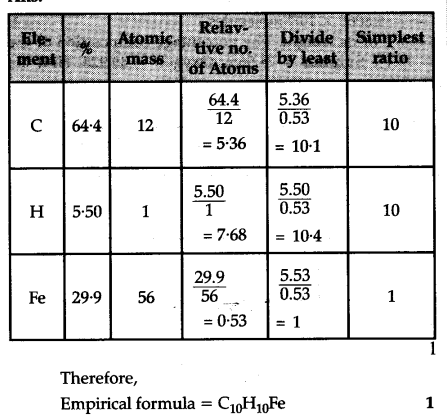# An organometallic compound on analysis was found to contain, C = 64.4%, H = 5.5% and Fe = 29.9%

An organometallic compound on analysis was found to contain, C = 64.4%, H = 5.5% and Fe = 29.9%. Determine its empirical formula.
(At mass of Fe = 564)